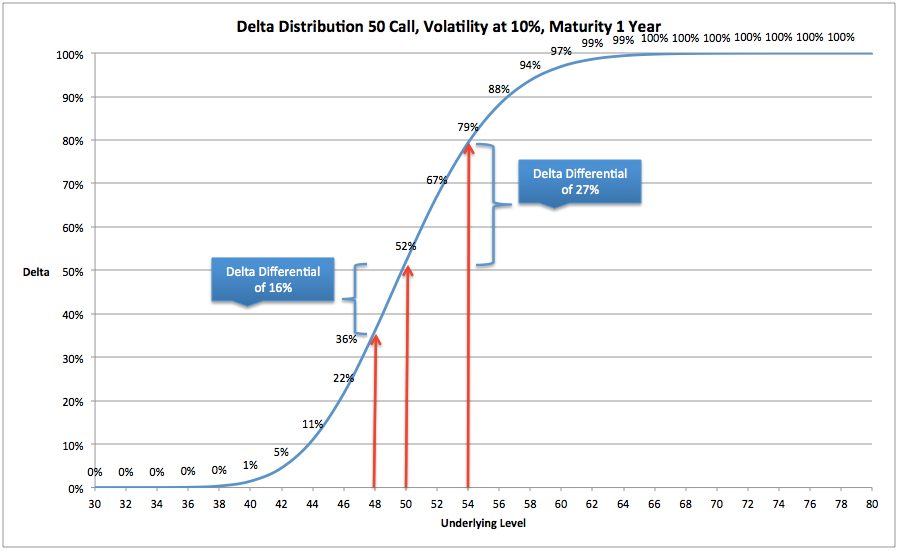# Options Trading V, Gamma Distribution in relation to VolatilityThis publication is part of a series of posts on the Greeks. These posts are a short summary of a part of the content of my book How to Calculate Options Prices and Their Greeks.

In options Trading IV, we’ve shown how the change of the Delta of an option (i.e. the Gamma) can be employed to generate a certain profit. Hedging these Delta changes is called Gamma trading.In the chart above is shown how the Delta of a 50 call changes at different levels in the underlying. The person being long this option can generate a very decent P&L. But, one will have to keep in mind that an option becomes less worth every day (the Time decay or Theta), so actually the Gamma long trader will have good optionality (the possibility of scalping the Underlying), but it will come at a cost (Theta). He hopes that the positive result of his Gamma trades will outweigh the cost of Time decay.

The Gamma short trader is aiming for the opposite. Being short Gamma, he will need to hedge his position by buying high and selling low and thus will end up with a negative P&L with regards to his Delta hedges. He, however, is betting that the Time decay, which he “earns” will outweigh the cost of his negative scalps.

What was discussed as well in my previous post is the feature that an option (for the around the at the money strikes) will get a steeper distribution of the Delta change when Volatility will be lower. A steeper Delta distribution implies a higher Gamma; the Delta, for the calls, grows from 0 to 100% (for puts 0 to -100%) in a much faster pace, as shown in the chart below where a comparison is made for the Delta distribution at 10% and at 20% Volatility.The next step is to construct a Gamma distribution, which is the derivative of the Delta. When having a look at the Delta distribution, one can see that when the Underlying is at a low level, let’s say 36, the Delta at 10% Volatility is around 0%, meaning that the 50 call has no value anymore. The 50 call should have no Delta anymore, because it cannot get cheaper (cheaper than no value implies a negative value) when the Underlying is at a level which is lower than 36. Hence the Delta for the 50 call will remain at 0% for any level in the Underlying below 36. And whenever the Delta doesn’t change anymore, the Gamma should be zero. So we’ve found the boundary for Gamma (at 10% Volatility) at 36, which is the same as the boundary for the Delta (see my post: The End of Optionality, the Boundaries).

At 70 in the Underlying, the Delta of the 50 call has grown to 100% and cannot increase anymore since a call is the right to buy a fixed amount of Underlying units (100%). As a result Gamma should be zero at 70 and at higher levels.

In between 36 and 70, the Delta is growing, with the fastest pace when the Underlying is around 50 (the distribution is the steepest thus the derivative will be the highest). So when philosophising about Gamma; when Volatility is at 10%, Maturity 1 year and Strike is 50, there will be no Gamma below 36, the Gamma will increase towards 50, where it will have its maximum value. Above 50, towards 70, the Gamma will be decreasing towards 0, where it will remain at any level in the Underlying above 70.

It will look as shown in the chart below:When further considering Gamma; in the example as shown above, the Delta of the 50 call will grow in a range from 36 to 70 from 0% to 100%. As a result, the surface area of the Gamma chart should be 100% or just 1 (the Delta cannot grow more than a total of 100%).

When we now would consider the Delta distribution when Volatility would be 20%, we can conclude that the boundaries are further away. At this Volatility the Delta will be growing from 0 to 100% in the range 28 to 90. A much larger area and hence the change of the Delta will be less steep, in other words the Gamma will be lower.

At the same time the surface area of the Gamma distribution should remain at 1 or 100%. As a result, when having a higher Volatility, the distribution will be in a much larger range, however its Gamma value around the at the money should then be lower as compared to the Gamma at 10% Volatility.

For lower Volatility levels we can conclude that the range for the Delta to grow from 0 to 100% will be much smaller and as a result the value of the Gamma around the at the money should be much larger to keep the total surface area stable at 100%.

Below is shown how the Gamma is distributed at three different Volatility levels:As a conclusion we can say that if Volatility is higher, the range in which the option has Gamma will be much larger, however, (to keep the surface area stable) for the around the at the money options, the Gamma will be much lower.

For at the money options: the higher the Volatility, the lower the Gamma

For out of the money options: the higher the Volatility, the higher the Gamma